# RBSE Maths Class 9 Chapter 10: Important Questions and Solutions

RBSE Maths Chapter 10 – Area of Triangles and Quadrilaterals Class 9 Important questions and solutions are available here. The important questions and solutions of Chapter 10 provided at BYJU’S have detailed explanations. Also, RBSE Class 9 solutions are given here to help the students understand all the concepts clearly and to score maximum marks.

Chapter 10 of the RBSE Class 9 Maths contains major important concepts related to triangles and quadrilaterals. Important questions for all the exercises are given here, which cover various problems that can be solved using related theorems and axioms of triangles, as well as of quadrilaterals. After practicing these important questions, students will be able to prove certain statements with a combination of two or more plane figures and their properties.

## RBSE Maths Chapter 10: Exercise 10.1 Textbook Important Questions and Solutions

Question 1: Draw the following figures, on the same base and between the same parallel lines:

(i) An obtuse angled triangle and a trapezium

(ii) A parallelogram and an isosceles triangle

(iii) A square and a parallelogram

(iv) A rectangle and a rhombus

(v) A rhombus and a parallelogram

Solution:

(i) ABCD is a trapezium and ADC is an obtuse triangle, both lie on the same base CD and between the same parallel lines AB and CD.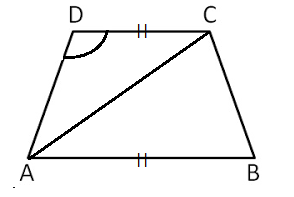(ii) ABCD is a parallelogram and AEB is an isosceles triangle, both lie on the same base AB and between the same parallel lines AB and CD.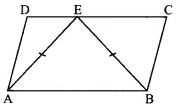(iii) ABCD is a square and CDEF is a parallelogram, both lie on the same base CD and have the same parallel lines.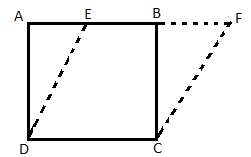(iv) ABCD is a rhombus and ABEF is a rectangle on the same base AB and between the same parallel lines AB and CF.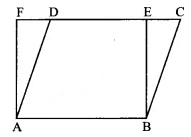(v) Two parallelograms ABCD and ABEF, both lie on the same base AB and between the same parallel lines AB and FC.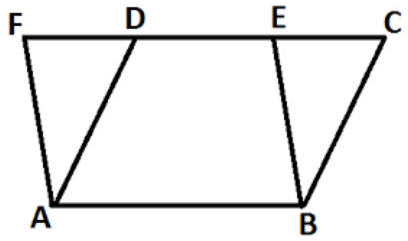## RBSE Maths Chapter 10: Exercise 10.2 Textbook Important Questions and Solutions

For more skill based questions on triangles and quadrilaterals students are suggested to visit here. Here, you can learn more about the area of triangles and quadrilateral in different situations with some practice questions.

## RBSE Maths Chapter 10: Exercise 10.3 Textbook Important Questions and Solutions

Question 2: ABCD is a parallelogram and X is the mid-point of AB. If ar(AXCD) = 24 cm², then ar (∆ABC) = 24 cm². Is this statement true? Give reason for your answer.

Solution:

Given,

ABCD is a parallelogram and X is the mid-point of AB.

Let us draw the diagonal AC.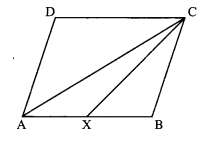We know that the diagonal of a parallelogram divides it into two triangles of equal areas.

Area of parallelogram ABCD = 2 x ar(∆ADC)

= 2 x 24

= 48 cm²

CX is the median of ∆ABC. (X is the midpoint of of AB)

ar(∆BCX) = 1/2 ar(∆ABC)

= (½) x 24

= 12 cm²

Now,

ar(∆XCD) = area of parallelogram ABCD – ar(∆BXC)

= 48 – 12

= 36 cm²

Hence, the given statement is false.

Question 3:

PQRS is a rectangle which is inside a quadrant of a circle of radius 13 cm. A is any point on PQ. If PS = 5 cm, then ar (∆PAS) = 30 cm². Is this statement true? Give reason for your answer.

Solution:

The given information can be represented as shown below.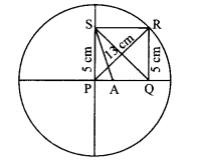In right ∆PQR,

By Pythagoras theorem,

PR² = PQ² + RQ²

PQ² = PR² – RQ²

= (13)² – (5)² (given PS = 5 cm, PR = 13 cm)

PQ = √(169-25)

= √144

PQ = 12 cm

Area of rectangle PQRS

= 12 x 5

= 60 cm²

We know that the diagonal of a rectangle bisects it into two triangles of equal areas.

Area of ∆PSQ = (½) x 60 = 30 cm²

Now, A is any point on PQ. So, the area of ∆APS depends on the position of A.

If A is at point Q, then the area will be 30 cm².

∴ ar (∆PAS) = 30 cm².

Hence, the given statement is true.

Question 4: PQRS is a parallelogram, whose area is 180 cm² and A is any point on the diagonal QS. Then the area of ∆ASR is 90 cm². Is this statement true? Give reason for your answer.

Solution:

Let us draw a parallelogram ABCD.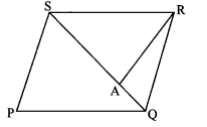Given,

Area of parallelogram PQRS = 180 cm²

QS is its diagonal which divides it into two triangles of equal areas.

∴ Area of ∆PQS = Area of ∆SRQ = 90 cm²

A is any point on QS. (given)

∴ Area of ∆ASR < Area of ∆SRQ

Hence, the given statement is false.

Question 5: In the figure, ABCD and EFGD are two parallelograms and G is the mid-point of the side CD. Show that: ar(∆DPC) = 1/2 ar(EFGD)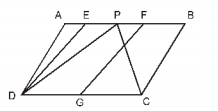Solution:

Given that, ABCD and EFGD are two parallelograms and G is the mid-point of a side CD.

∆PDC and parallelogram ABCD are on the the same base DC and between the same parallel lines AB and CD

ar(∆DPC) = 1/2 (area of parallelogram ABCD)

DG = 1/2 DC (G is mid-point of DC)

∴ ar(EFGD) = 1/2 ar(ABCD)

=> ar(∆DPC) = ar(EFGD)

Hence, the given statement can not be true.

Question 6: In a trapezium ABCD, AB || DC and L is the mid-point of BC. A line PQ || AD is drawn through L which meets AB on P and extended DC at Q as shown in the figure. Prove that: ar(ABCD) = ar(APQD)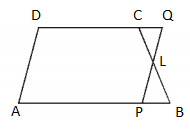Solution: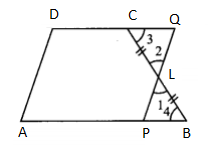In ∆LPB and ∆CLQ

∠1 = ∠2 (vertically opposite angles)

∠3 = ∠4 (alternate interior angles)

and BL = LC (L is mid-point of BC)

By ASA congruence criterion,

∴∆LPB ≅ ∆LQC

We know that congruent triangles have equal area.

⇒ ar(∆LPB) ≅ ar(∆LQC)….(i)

Now,

ar(ABCD) = ar(APQD) – ar(∆CLQ) + ar(∆BLP)

ar(ABCD) = ar(APQD) – ar(∆BLP) + ar(∆BLP)

ar(ABCD) = ar(APQD)

Hence proved.

Question 7: If the mid-points of any quadrilateral are joined in an order, then prove that the area such obtained parallelogram is half of the area of the given quadrilateral (see in the figure).

[Hint: Join BD and draw perpendicular from A on BD]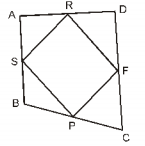Solution:

Join BD and BR.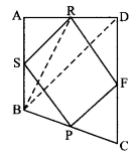Median BR divides ∆BDA into two triangles of equal areas.

ar(∆BRA) = 1/2 ar(∆BDA) ….(i)

Similarly, median RS divides ∆BRA into two triangles of equal areas.

ar(∆ASR) = 1/2 ar(∆BRA) ….(ii)

From equation (i) and (ii),

ar(∆ASR) = 1/4 ar(∆BDA) ….(iii)

Similarly,

ar(∆CFP) = 1/4 ar(∆BCD) ….(iv)

ar(∆ASR) + ar(∆CFP) = 1/4 [ar(∆BDA) + ar(∆BCD)]

ar(∆ASR) + ar(∆CFP) = 1/4 ar(quadrilateral ABCD) ….(v)

Similarly, ar(∆DRF) + ar(∆BSP) = 1/2 ar(quadrilateral ABCD) ….(vi)

ar(∆ASR) + ar(∆CFP) + ar(∆DRF) + ar(ABSP) = 1/2 ar(quadrilateral ABCD) ….(vii)

But

ar(∆ASR) + ar(∆CFP) + ar(∆DRF) + ar(∆BSP) + ar(parallelogram PFRS)

Subtracting (vii) from (viii),

ar(parallelogram PFRS) = ½ ar(quadrilateral ABCD)

Hence proved.

Question 8: A man walks 10 m in the East and then 30 m in the North side. Find his distance from the initial point.

Solution:

The given information can be shown diagrammatically as below.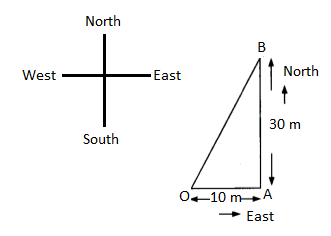In right triangle ABC,

By Pythagoras theorem,

OB2 = OA2 + AB2

= 102 + 302

= 100 + 900

= 1000

OB = √1000

OB = 10√10 m

Therefore, the distance from the initial point is 10√10 m.

Question 9: A ladder is placed with a wall such that its lower end at a distance from the wall is 7 m. If its other end reaches a window height of 24 m, then find the length of the ladder.

Solution:

The given information can be shown diagrammatically as below.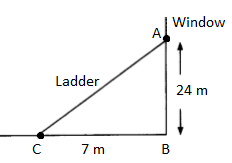In right ∆ABC,

By Pythagoras theorem,

AC² = AB² + BC²

AC² = (24)² + (7)²

= 576 + 49

= 625

=> AC = √625 = 25 m

Therefore, the length of the ladder = 25 m

Question 10: Two poles of height 7m and 12 m are standing on a plane ground. If the distance between their feet is 12 m, then find the distance between upper ends of poles.

Solution:

The given information can be shown diagrammatically as below.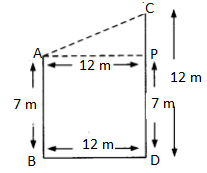AB = 7 m and CD = 12 m

CP = (12 – 7) m = 5 m

∴In right triangle APC,

By Pythagoras theorem,

AC² = AP² + PC²

= (12 m)² + (5 m)²

= 144 m² + 25 m²

=> AC = √169 = 13 m

Therefore, the distance between their upper ends is 13 m.

Question 11: Find the length of altitude and area of an equilateral triangle whose length of side is a.

Solution:

Let ABC is an equilateral triangle.

AB = BC = CA = a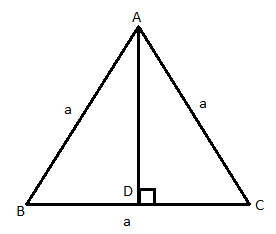AD is the altitude of the triangle.

BD = 1/2 BC = a/2

In right triangle ABD,

= a2 – (a/2)2

= a2 – a2/4

= (4a2 – a2)/4

= 3a2/4

Therefore, length of the altitude = AD = (√3)a/2 units

Area of triangle ABC = 1/2 × (BC) × (AD)

= (1/2) × a × (√3)a/2)

= (√3)a2/4 sq. units

Question 12: Find the length of diagonal of a square whose each side is 4 m.

Solution:

Let the side of a square = a = 4 m

Length of diagonal of a square = √2a

= √2 × 4

= 4√2 m

Question 13: If an equilateral triangle ABC, AD is perpendicular on BC then prove that 3AB2 = 3AD2.

Solution:

In an equilateral triangle ABC, AD ⊥ BC.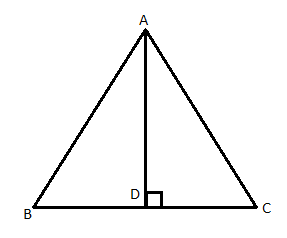AB = BC = CA

BD = ½ BC

In right triangle ABD,

AB2 = 1/4 AB2 + AD2

AB2 – 1/4 AB2 = AD2

Hence proved.

Question 14: O is any point inside a rectangle ABCD. Prove that: OB2 + OD2 = OA2 + OC2

Solution:

In the rectangle ABCD, join O, with the vertices A, B, C and D also, draw a parallel line LM through O which meets AB and DC at M and L, respectively.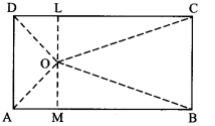In right ∆OMB,

OB² = OM² + MB²

OB² = OM² + CL² (by Pythagoras theorem)

ABCD is a rectangle then ML ⊥ AB.

MB = CL

In right ∆ODL,

OD² = OL² + DL²

= OL² + AM² (∵ DL = AM)

∴ OB² + OD² = OM² + CL² + OL² + AM²

= (OM² + AM²) + (CL² + OL²)

= OA² + OC²

That is, OB² + OD² = OA² + OC²

Hence proved.

Question 15: In an obtuse angled triangle ABC, ∠C is an obtuse angle. AD ⊥ BC meets BC at D on extending forward. Prove that: AB² = AC² + BC² + 2BC × CD

Solution:

Let us draw an obtuse angled triangle ABC as shown below.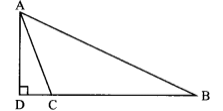AC² = AD² + DC² ….(i)

AB² = AD² + (DC + CB)²

AB² = AD² + DC² + BC² + 2BC × DC

AB² = (AD² + DC²) + BC² + 2BC × CD

AB² = AC² + BC² + 2BC × CD [From (i)]

Hence proved.

### RBSE Maths Chapter 10: Additional Important Questions and Solutions

Question 1: Median of a triangle divides it into two:

(a) triangles of equal areas

(b) congruent triangles

(c) right angled triangles

(d) isosceles triangles

Solution:

The median on the base of a triangle can be formed by joining the midpoint of the base to the opposite vertex. It results in two triangles with equal bases (half of the original triangle) and with the same altitude (one triangle will have altitude inside it whereas another having outside of it) whose areas will be the same.

Question 2: In which of the following figures, you find two polygons made on the same base and between the same parallels?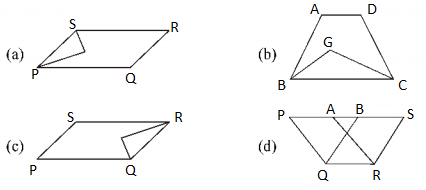Solution:

In the figure (d), two polygons are made on the same QR and between the same parallel lines QR and PS.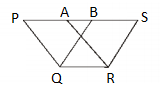Question 3: The figure made by joining the mid-points of adjacent sides 8 cm and 6 cm of a rectangle is:

(a) a rectangle of area 24 cm2

(b) a square of area 25 cm2

(c) a trapezium of area 24 cm2

(d) a rhombus of area 24 cm2

Solution:

We know that line segments joining the mid-points of sides of a rectangle form a rhombus.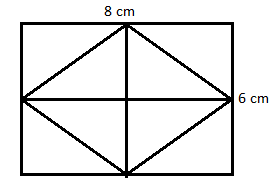Area of rhombus = ½ (product of two diagonals)

= 1/2 × 8 × 6

= 24 cm2

Question 4: In the figure, area of parallelogram ABCD is: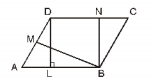(a) AB x BM

(b) BC x BN

(c) DC x DL

Solution:

Area of parallelogram = base × height

= AB × DL

Or

= DC × DL

Or

= AB × BN or DC × BN

Question 5: In the figure, if parallelogram ABCD and rectangle ABEM are of equal areas, then: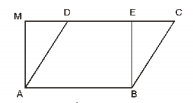(a) perimeter of ABCD = perimeter of ABEM

(b) perimeter of ABCD < perimeter of ABEM+

(c) perimeter of ABCD > perimeter of ABEM

(d) perimeter of ABCD = 1/2 (perimeter of ABEM)

Solution:

In rectangle ABEM, AB = EM ….(i)[sides of rectangle]

In parallelogram ABCD, CD = AB ….(ii)

AB + CD = EM + AB …(iii)

We know that the perpendicular distance between two parallel sides of a parallelogram is always less than the length of the other parallel sides.

BE < BC and AM < AD (In a right triangle, the hypotenuse is the largest side)

BC + AD> BE + AM

Adding AB + CD both sides,

AB + CD + BC + AD> AB + CD + BE + AM

⇒ AB + BC + CD + AD> AB + BE + EM + AM (∴ CD = AB = EM)

Perimeter of parallelogram ABCD > Perimeter of rectangle ABEM

Question 6: Mid-points of the sides of a triangle ABC make a simple quadrilateral by taking with any vertex as fourth point whose area is equal to:

(a) 1/2 ar(∆ABC)

(b) 1/3 ar(∆ABC)

(c) 1/4 ar(∆ABC)

(d) ar(∆ABC)

Solution:

Let D, E and F be the midpoint of sides AB, BC and CA, respectively.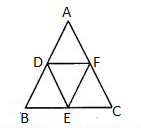ar(ΔADF)= ar(ΔDBE) = ar(ΔECF) = ar(ΔDEF) ….(i)

ar(ΔDEF) = 1/4 ar(ΔABC) ….(ii)

If E is taken as the fourth vertex, then area of quadrilateral ADEF

= 2 ar(ΔDEF) [From (i)]

= 2 × 1/4 ar(ΔABC) [From (ii)]

= 1/2 ar(ΔABC)

Question 7: Two parallelograms are on the same bases and between the same parallel lines. Ratio of their areas is:

(a) 1 : 2

(b) 1 : 1

(c) 2 : 1

(d) 3 : 1

Solution:

Area of parallelogram = base × height

The distance between two parallel lines is always equal.

Given that, two parallelograms are on the same base and between the same parallel lines.

That means the base and heights of the parallelograms are equal.

Hence, their areas are also equal.

Therefore, ratio of their areas = 1 : 1

Question 8: A triangle and a parallelogram are on the same base and between same parallel lines, then the ratio of areas of the triangle to the area of parallelogram is:

(a) 1 : 3

(b) 1 : 2

(c) 3 : 1

(d) 1 : 4

Solution:

If a triangle and a parallelogram are on the same base and between the same parallel lines, then the area of the triangle is equal to half the area of the parallelogram.

area of triangle = 1/2 area of parallelogram

Therefore,

area of triangle : area of parallelogram = 1 : 2

Question 9: ABCD is a trapezium with sides AB = a cm and DC = b cm (in the figure). E and F are midpoints of non-parallel sides. Ratio of ar(ABFE) and ar(EFCD) is: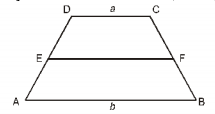(a) a : b

(b) (3a + b) : (a + 3b)

(c) (a + 3b) : (3a + b)

(d) (2a + b) : (3a + b)

Solution:

From the given figure,

EF = (AB + CD)/2 = (a + b)/2

Let h be the height of trapezium ABFE and EFCD.

Area of trapezium = 1/2 × (sum of two parallel sides) × height

ar(ABFE) : ar(EFCD) = 1/2 [a + (a + b)/2] × h : 1/2 [b + (a + b)/2] × h

= (2a + a + b)/2 : (2b + a + b)/2

= (3a + b) : (a + 3b)

Question 10: In the figure, PSDA is a parallelogram. Points Q and R are on PS are taken such that PQ = QR = RS and PA || QB || RC. Prove that: ar(∆PQE) = ar(∆CFD)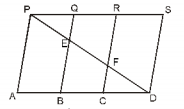Solution:

Given,

PSDA is a parallelogram.

PQ = QR = RS and PQ || QB || RC

PQ || AB (given PS || AD)

and PA || QB (given)

Thus, PABQ is a parallelogram.

PQ = AB ….(i)

Similarly, QBCR is also a parallelogram.

QR = BC ….(ii)

RCDS is also a parallelogram.

RS = CD ….(iii)

PQ = QR = RS ….(iv) (given)

From (i), (ii), (iii) and (iv),

PQ = QR = RS = AB = BC = CD ….(v)

In ∆PQE and ∆DCF,

∠QPE = ∠FDC (alternate interior angle)

PQ = CD [From (v)]

∠PQE = ∠FCD

∠PQE = ∠QBC (alternate interior angles)

and ∠QBC = ∠FCD (corresponding angles)

By ASA congruence criterion,

∆PQE ≅ ∆DCF

We know that the areas of two congruent figures are the same.

Therefore, ar (∆PQE) = ar (∆DCF)

Hence proved.

Question 11: X and Y are two points on the side LN of the ∆LMN such that LX = XY = YN. Through X, a line is drawn parallel to LM which meets MN at Z (see in the figure). Prove that: ar(∆LZY) = ar(quadrilateral MZYX)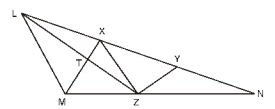Solution:

Given,

X and Y are the points on side LN of ∆LMN, such that LX = XY = YN and LM || XZ.

Here, ∆XMZ and ∆ZLX are on the same base XZ and between the same parallel LM and XZ.

Thus, ar(∆XMZ) = ar(∆ZLX) ….(i)

Adding ar(∆XYZ) on both sides of equation (i),

ar(∆XMZ) + ar(∆XYZ) = ar(∆ZLX) + ar(∆XYZ)

Hence proved.

Question 12: Area of parallelogram ABCD is 90 cm2 (see in the figure). Find the area of

(i) ar(ABEF)

(ii) ar(ABD)

(iii) ar(BEF)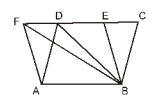Solution:

Given,

Area of parallelogram ABCD = 90 cm2

(i) We know that parallelograms on the same base and between the same parallel lines have equal area.

ar(parallelogram ABEF) = ar(parallelogram ABCD) = 90 cm2

(ii) We know that, if a triangle and a parallelogram are on the same base and between the same

parallels, then the area of the triangle is equal to half of the area of the parallelogram.

In the figure, parallelograms lie on the same base AB and between the same parallels AB and EF.

ar(∆ABD) = 1/2 ar(parallelogram ABEF)

= 1/2 x 90

ar(∆ABD) = 45 cm2

(iii) Similarly, triangle BEF and parallelogram ABEF are on the same base EF and EF || AB.

ar(∆BEF) = 1/2 ar(parallelogram ABEF)

= 1/2 x 90

= 45 cm2

Question 13: In ∆ABC, D is the mid-point of side AB and P is any point on side BC. If line segment CQ ∥ PD meets side AB at Q (see in the figure), then prove that: ar(∆BPQ) = 1/2 ar(∆ABC)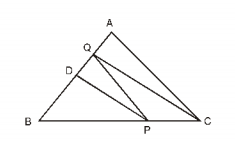Solution:

Given,

In ∆ABC, D is the mid-point of AB.

CQ || PD and CQ intersects AB at Q.

Join CD.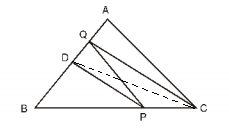Since D is the mid-point of AB. So, on ∆ABC, CD is the median.

Then, ar(∆BCD)= 1/2 ar(∆ABC) ….(i)

Here, ∆PDQ and ∆PDC are on the same base PD and between the same parallels PD and CQ.

Thus, ar(∆BCD) = 1/2 ar(∆ABC) [From (i)]

ar(∆BDP) + ar(∆DC) = 1/2 ar(∆ABC)

ar(∆BDP) + ar(∆PDQ) = 1/2 ar(∆ABC)

ar(∆BPQ) = 1/2 ar(∆ABC)

Hence proved.

Question 14: ABCD is a square. E and F are respectively mid-points of sides BC and CD. If R is the mid-point of line segment EF(see in the figure), then prove that: ar(∆AER) = ar(∆AFR)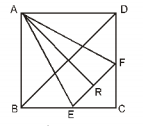Solution:

Given,

ABCD is a square

AB = BC = CD = DA

E and F are mid-points of BC and CD, respectively.

BE = DF

By SAS congruence rule,

⇒ AE = EF (by CPCT)

Therefore, ∆AEF is an isosceles triangle.

R is mid-point of EF.

⇒ ∆ARE ≅ ∆ARF (∵ AE = AF, EF = RF, ∠ARE = ∠ARF = 90°)

We know that the areas of two congruent figures have equal area.

⇒ ar(∆AER) = ar(∆AFR)

Hence proved.

Question 15: O is any point on diagonal PR of a parallelogram PQRS (see in the figure). Prove that: ar(∆PSO) = ar(∆PQO)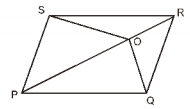Solution:

Given,

PQRS is a parallelogram and O is any point on the diagonal PR.

Join QS which intersect PR at B.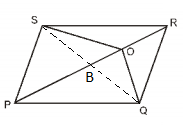We know that the diagonals of a parallelogram bisect each other.

B is the mid-point of SQ.

Therefore, PB is a median of ∆QPS.

Median of a triangle divides it into two triangles of equal area.

Thus, ar(∆BPQ) = ar(∆BPS) ….(i)

Also, OB is a median of ∆OSQ.

ar(∆OBQ) = ar(∆OBS) ….(ii)

ar(∆BPQ) + ar(∆OBQ) = ar(∆BPS) + ar(∆OSB)

⇒ ar(∆PQO) = ar(∆PSO)

Hence proved.

Question 16: ABCD is a parallelogram in which side BC is extended upto point E such that CE = BC (see in the figure). AE intersects the CD at F. If ar(∆DFB) = 3 cm2, then find the area of parallelogram ABCD.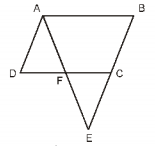Solution:

Given,

ABCD is a parallelogram and CE = BC.

That means, C is the mid-point of BE.

Join BD and BF.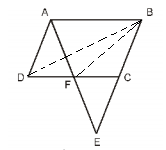Now, ar(∆DFB) = 3 cm2 (given)

We know that ∆DFB and ∆ADF are on the same base DF and between the same parallels AB and DF.

Therefore, ar (∆ADF) = ar (∆DFB) = 3 cm2 ….(i)

In ∆ABE,

By converse of mid-point theorem,

EF = AF ….(ii) (given C is the mid-point of BE)

∠AFD = ∠CFE (vertically opposite angles)

AF = EF [from equation (ii)]

and ∠DAF = ∠CFE (alternate interior angles)

By ASA congruence rule,

⇒ ar(∆ADF) = ar(∆CFE) (since the areas of congruent figures are equal)

ar(∆CFE) = ar(∆ADF) = 3 cm2 ….(iii) [From (i)]

Now, in ∆BFE, C is the mid-point of BE, i.e. CF is the median of ∆BFE.

We know that the median of a triangle divides it into two triangles of equal areas.

ar(∆CFE) = ar(∆BFC)

From (iii),

ar(∆BFC) = 3 cm2 ….(iv)

Now,

ar(∆BDC) = ar(∆DFB) + ar(∆BFC)

= 3 + 3 [from (i) and (iv)]

= 6 cm2

In parallelogram ABCD,

ar(∆ABD) = ar(∆BDC)

(diagonal of a parallelogram divides it into two congruent triangles of equal areas)

Or

ar(∆BDC) = 1/2 ar(parallelogram ABCD)

ar(parallelogram ABCD) = 2 ar(∆ABC)

= 2 x 6

= 12 cm2

Hence, the area of parallelogram ABCD = 12 cm2

Question 17: Point E is taken on side BC of a parallelogram ABCD. AE and DC are extended so that they meet at F. Prove that: ar(∆ADF) = ar(∆BFC)

Solution:

Given information can be drawn as shown below.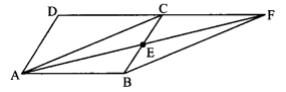ar(parallelogram ABCD) = ar(parallelogram ABFC) ….(i)

ar(∆ABF) = ar(∆AFC)

ar(∆AFC) = 1/2 ar (ABCD) ….(ii)

Also,

From (ii) and (iii),

= ar(∆BCD) + ar(∆BFC)

= ar(∆BFC) + ar(∆ABC)

Hence proved.

Question 18: Diagonals of a parallelogram ABCD bisect at O. A line is drawn through O which meets AD at P and BC at Q. Show that PQ divides ABCD into two parts equal in area.

Solution:

Given,

ABCD is a parallelogram in which AC and BD meet at O.

A line is drawn through O which meets AD at P and BC at Q.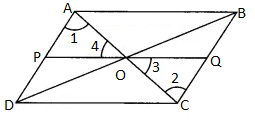AC is a diagonal of parallelogram ABCD.

Also, ∠1 = ∠2, ∠4 = ∠3 and OA = OC

Thus, ∆OAP ≅ ∆OQC (by ASA congruence rule)

⇒ ar(∆OAP) = ar(∆OQC) ….(ii)

Subtracting (ii) from (i),

ar(∆ADC) – ar(∆OAP) = ar (∆ABC) – ar (∆OQC)

⇒ ar (OPDC) = ar (AOQB)

⇒ ar (OPDC) + ar (AOQC) = ar (AOQB) + ar (OAP)

⇒ ar (PQCD) = ar (ABQP)

Hence proved.

Question 19: Medians BE and CF of a ∆ABC intersect each other at point G. Prove that the area of ∆GBC is equal to the area of quadrilateral AFGE.

Solution:

Given,

Medians BE and CF of a ∆ABC intersect each other at point G.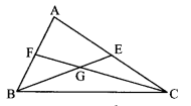Since, CF is the median, then

ar (∆AFC) = ar (∆CBF)

ar (∆AFC) = 1/2 ar (∆ABC) ….(i)

And

and ar (∆BEC)= 1/2 ar (∆ABC) ….(ii)

From (i) and (ii),

ar (∆AFC) = ar (∆BEC)

Subtracting ar (∆GEC) from both sides,

ar (∆AFC) – ar (∆GEC) = ar (∆BEC) – ar (∆GEC)

ar (AFGE) = ar (∆BGC)

Hence proved.

Question 20: In the figure, CD || AE and CY || BA. Prove that: ar (∆CBX) = ar (∆AXY)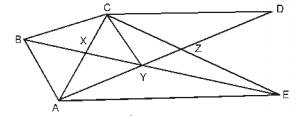Solution:

Given that, CD || AE and CY || BE.

In the given figure, ∆ABC and ∆BAY lie on the same base AB and between the same parallels AB and CY.

ar (∆ABC) = (∆BAY)

ar (∆ABX) + ar (∆CBX) = ar (∆ABX) + ar (∆AXY)

ar (∆CBX) = ar (∆AXY)

Hence proved.

Question 21: ABCD is a trapezium in which AB || CD, CD = 30 cm and AB = 50 cm. If X and Y are mid-points of AD and BC respectively, then prove that: ar (DCYX) = 7/9 ar (XYBA)

Solution:

According to the given,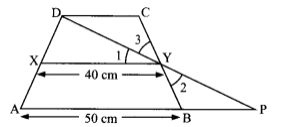In ∆DCY and ∆PBY,

CY = BY (given)

∠C = ∠B (alternate interior angles)

and ∠3 = ∠2 (vertically opposite angles)

By ASA congruence rule,

∆DCY ≅ ∆PBY

Then DC = BP (by CPCT)

⇒ DC = PB = 30 cm

AP = BA + BP = 50 + 30 = 80 cm

Using mid-point theorem,

XY = 1/2 AP

= 1/2 x 80

= 40 cm

Let the distance between AB, XY and DC be h cm.

In trapezium DCYX,

ar (trapezium DCYX)

= 1/2 h(30 + 40)

= 1/2 (70 h)

= 35h cm2

ar(trapezium XYBA) = 1/2 h(40 + 50)

= 1/2 (90h)

= 45h cm2

Now,

ar(DCYX)/ar(XYBA) = 35/45

ar(DCYX)/ar(XYBA) = 7/9

ar(DCYX) = 7/9 ar(XYBA)

Hence proved.

Question 22: In ∆ABC, L and M are the points on sides AB and AC, respectively such that LM || BC. Also, LC and BM intersect at O. Prove that ar (∆LOB) = ar (∆MOC).

Solution:

Given,

In ∆ABC, L and M are the points on sides AB and AC and LM || BC.

Also, LC and BM intersect each other at O.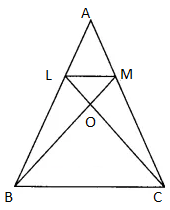Here, ∆LBC and ∆MBC both lie on the same base BC and between the same parallel lines BC and LM.

Thus, ar (∆LBC) = ar (∆MBC)

⇒ ar (∆LOB) + ar (∆BOC) = ar(∆MOC) + ar(∆BOC)

⇒ ar (∆LOB) = ar (∆MOC)

Hence proved.

Question 23: In the figure, ABCDE is a pentagon. BP drawn parallel to AC, meets extended DC at P and EQ drawn parallel to AD meets extended CD at Q. Prove that: ar (ABCDE) = ar (∆APQ)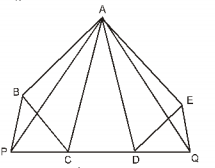Solution:

Given,

ABCDE is a pentagon, BP || AC and EQ || AD.

We know that triangles on the same base and between the same parallel lines have equal areas.

Similarly, ar (∆CAB) = ar (∆CAP) ….(ii) (same base is AC and AC || BP)

ar (∆ADE) + ar (∆CBA) + ar (∆CDA) = ar (∆ADQ) + ar (∆CAP) + ar (∆CDA)

⇒ ar (ABCDE) = ar (∆APQ)

Hence proved.

Question 24: If medians of a triangle ABC meet at point G, then prove that: ar (∆AGB) = ar (∆AGC) = ar (∆BGC) = 1/3 ar (∆ABC)

Solution:

Let AD, BE and CF be the medians of triangle ABC which intersect at G.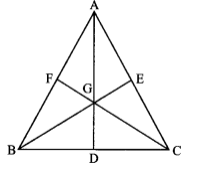We know that the median of a triangle divides it into two triangles of equal areas.

In ∆ABC, AD is a median.

ar (∆ABD) = ar (∆ACD) ….(i)

In ∆CBG, GD is a median.

ar (∆GBD) = ar (∆GCD) ….(ii)

Subtracting (ii) from (i),

ar (∆ABD) – ar (∆GBD) = ar (∆ACD) – ar (∆GCD)

⇒ ar (∆AGB) = ar (∆AGC)

Similarly,

ar (∆AGB) = ar (∆BGC)

ar (∆AGB) = ar (∆AGC) = ar (∆BGC) ….(iii)

Now,

ar (∆ABC) = ar (∆AGB) + ar (∆AGC) + ar (∆BGC) = 3 ar (∆AGB) [From (iii)]

ar (∆AGB) = 1/3 ar (∆ABC)

Therefore, ar (∆AGB) = ar (∆AGC) = ar (∆BGC) = 1/3 ar (∆ABC)

Hence proved.

Question 25: In the figure, X and Y are respectively the mid-points of sides AC and AB. QP || BC and CYQ and BXP are straight lines. Show that ar (ABP) = ar (ACQ)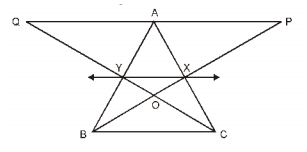Solution:

Given,

X and Y are mid-point of sides AC and AB, respectively.

And XY || BC.

In the given figure, triangles BYC and BXC are on the same base BC and lie in between the same parallel lines BC and XY.

ar (∆BYC) = ar (∆BXC)

Subtracting ar (∆BOC) from both the sides,

⇒ ar (∆BYC) – ar (∆BOC) = ar (∆BXC) – ar (∆BOC)

⇒ ar (∆BOY) = ar (∆COX)

Adding ar (∆XOY) on both the sides,

⇒ ar (∆BOY) + ar (∆XOY) = ar (∆COX) + ar (∆XOY)

⇒ ar (∆BXY) = ar (∆CXY) ….(i)

Similarly, quadrilaterals XYAP and XAQY are on the same base XY and between the same parallels XY and PQ.

ar (∆BXY) + ar (quadrilateral XYAP) = ar (∆CXY) + ar (quadrilateral XYQA)

Therefore, ar (∆ABP) = ar (∆ACQ)

Question 26: In the figure, ABCD and AEFD are two parallelograms. Prove that: ar(PEA) = ar(QFD). [Hint: Join PD]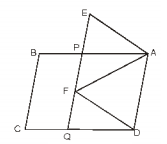Solution:

Given that, ABCD and AEFD are two parallelograms.

In parallelogram ABCD, AB || CD.

In parallelogram AEFD, FE || AD.

AP || DQ

Thus, PQDA is a parallelogram.

Also, parallelograms PQDA and AEFD are on the same base AD and between the same parallels AD and EQ.

ar (parallelogram PQDA) = ar (parallelogram AEFD)

Subtracting ar (quadrilateral APFD) from both sides,

ar (parallelogram PQDA) – ar (quadrilateral APFD)

= ar (parallelogram AEFD) – ar (quadrilateral APFD)

⇒ ar (∆QFD) = ar (∆PEA)

Hence proved.